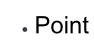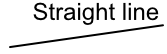Geometrical Terms

## Geometrical TermsA point is a specific location on a diagram or on a graph. It may be shown as a dot, given as a measurement or as a set of co-ordinates.A line is a path between two points. A straight line is the shortest distance between two point. A line has a length.Parallel lines are two, straight lines that always stay the same distance apart. The lines never meet.An angle is the measurement of the turn between two intersecting lines. Angles are measured in degrees.An angle of 90 degrees is a right angle. Where one line intersects another line at a right angle, the lines are perpendicular to each other.

## Example 1

What is an angle of 90º called?

A Right Angle is 90º. It is shown as a square on diagrams, rather than as a curve.